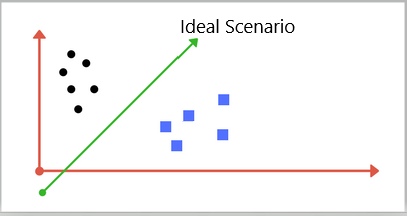# Support Vector Machine

## What are Support Vector Machines?

A support vector machine is a collection of supervised learning algorithms that use hyperplane

graphing to analyze new, unlabeled data. These machines are mostly employed for classification problems, but can also be used for regression modeling.

## How are Support Vector Machines Used?

In the most common application, incoming data is categorized into one of two classes. For SVM models, each data point is interpreted as a p-dimensional vector, which the machine attempts to create a linear classifier by fitting the data point inside a hyperplane (p-1 dimension).

So for classification, every input is turned into a point in n-dimensional space (n being the number of features), and the value of each feature defined as the value of a unique coordinate on the hyperplane. Classification is determined by finding the hyperplane that most clearly separates the two classes. For example: